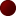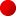uncompiled
stopped

BlinkenCPU simulates an array of bits which are mangled by a simple assembly-like program input by the user. In one way, BlinkenCPU is a system for creating cellular automata. In another, it's a very simple parallel computer.

Visualize each light as a simple CPU. It can perform several simple computational tasks by using its value and the value of one of its neighbors. By combining these simple tasks, interesting displays can be created.

By clicking on the lights in the array, you can toggle their value between 1 (which shows up as a lit LED), and 0 (which is an unlit LED). Most operations on the lights (also commonly called cells) will give them either a 1 or 0 value, but they can hold any numerical value. A cell will be lit if its value is non-zero, and unlit if its value is zero.

The BlinkenCPU language is a simple assembly-like language with logical operators (AND, OR, NOT, XOR), arithmetic (ADD, SUB, INC, DEC), comparators (GTI, LTI, EQI, NEI), and memory operators (STO, RCL, SWP, ZERO). Each cell has an accumulator that stores the result of computation, and a temporary scratch memory space that can store one value.

AND, OR, XOR, ADD, and SUB all take a memory reference, designated with one of the eight directional identifiers: N, S, E, W, NE, NW, SE, SW, and the special identifier O, referring to the scratch memory space. Directional references loop around edges. Each of these operators computes the accumulator against the given memory reference, storing the result in the accumulator. For example, ADD W sums the accumulator and the value of the cell to the west, and stores the result in the accumulator.

NOT logically inverts the accumulator value, and ZERO stores a zero in the accumulator. INC and DEC increment and decrement the accumulator value. GTI, LTI, EQI, and NEI compare the accumulator with an immediate value, storing the logical result in the accumulator. STO stores the accumulator in the scratch storage memory, RCL loads the accumulator from the scratch storage memory, and SWP swaps the values in the accumulator and the scratch storage memory.

A simple example:

```xor e
or n
```

This small program takes the XOR of each cell with its left neighbor, and then ORs that with the value of the upper neighbor. Light one cell, then run this program to see an interesting result. :)

The language is case-insensitive, and only recognizes the first two words on a line. The rest of the line may be used for comments. Blank lines and lines beginning with a semicolon (;) are ignored. Once the program is entered, click 'compile' to load it into the BlinkenCPU, then use 'run,' 'stop,' and 'step' to compute. 'Reset' will clear the cell data, but leave the program intact.

## BlinkenCPU Computational Model

The program entered will be run once per time step on each cell. At the beginning of computation, the accumulator for each cell is initialized with the current value of the cell. Memory references to neighboring cells and the current cell are read from their values in the previous time step. In this way, computation results for the same time step in adjacent cells cannot affect the current computation. The value in the accumulator at the end of computation will become the value of the cell for the next time step. It may be useful to think of the accumulator as a temporary scratch space where computation is done before updating the actual value of the cell.

In addition to spatial memory reference, there is one memory storage location manipulated with the STO, RCL, and SWP instructions. Unlike spatial memory, this storage location is updated as computation proceeds, and can thus be used as temporary computational scratch space.

While the facilities provided may seem spartan, they provide enough functionality to compute Conway's Game of Life. (The implementation of Conway's Game of Life is left as an exercise to the reader)

## Instruction Reference

In the following terse descriptions, ACC is the accumulator, MEM is a memory reference, and STORAGE is the scratch storage unit.

### Logical operators

AND MEM: ACC ← ACC ∧ MEM

OR MEM: ACC ← ACC ∨ MEM

XOR MEM: ACC ← ACC ⊕ MEM

NOT: ACC ← ¬ ACC

### Arithmetic

ADD MEM: ACC ← ACC + MEM

SUB MEM: ACC ← ACC - MEM

INC: ACC ← ACC + 1

DEC: ACC ← ACC - 1

### Comparators

GTI VALUE: ACC ← 1 if ACC > VALUE, 0 otherwise

LTI VALUE: ACC ← 1 if ACC < VALUE, 0 otherwise

EQI VALUE: ACC ← 1 if ACC = VALUE, 0 otherwise

NEI VALUE: ACC ← 1 if ACC ≠, 0 otherwise

### Memory operators

STO: STORAGE ← ACC

RCL: ACC ← STORAGE

SWP: Swap ACC and STORAGE

ZERO: ACC ← 0